• 联合概率分布P（x,y）进行建模，即特征x和标记y共同出现的概率。通过贝叶斯公式求得使p（yi|x）值最大的yi。其本质是联合概率分布可以理解为“生成”（X,Y）样本的依据。已知X，从Y的候选集中任选一个，可能有（X...
判定模型与生成模型

判定模型

对条件概率分布P（y|x）进行建模，即在特征x出现的情况下标记y出现的概率。其本质是根据X判定Y。

举例：若要确定一只羊是山羊还是绵羊，需从历史数据中训练得到判定模型，通过提取这只羊的特征来判断这只羊是山羊的概率大还是绵羊的概率。

生成模型

对联合概率分布P（x,y）进行建模，即特征x和标记y共同出现的概率。通过贝叶斯公式求得使p（yi|x）值最大的yi。其本质是联合概率分布可以理解为“生成”（X,Y）样本的依据。已知X，从Y的候选集中任选一个，可能有（X，Y1）,…,(X,Yn)，鉴于实际数据的“生成”方式依赖P（x,y），故我们选择概率最大者最为结果。

举例：若要确定一只羊是山羊还是绵羊，需根据山羊的特征训练出山羊模型，并根据绵羊的特征训练出绵羊模型，然后从这只羊中提取特征后依次与两个模型进行比对，结果为概率大者。

自编码器中的Decoder属于生成模型。

高效的数据表示

以下两组数据，哪组更容易记忆？

40，27，25，36，81，57，10，73，19，68

50，25，76，38，19，58，29，88，44，22，11，34，17，52，26，13，40，20

尽管第一序列比第二序列短，但第二序列有明显规律。如果能够发现其规律，则除记住规律只需记住其第一个数字及序列长度即可，故第二序列更容易记忆。但假设某人记忆力超群，他可能不会关心数字序列是否存在规律。因此我们必须对自编码器增加约束以强制其探索数据中的规律。

注：

此组数据为希尔顿序列，其规则是：偶数后一数字的值是其二分之一，奇数后一数字的值是其三倍加一。

什么是自编码器

自编码器（AutoEncoder，AE）是旨在使输出特征近似等于输入标记的自监督学习神经网络。它借助稀疏编码的思想，捕捉并使用原始输入特征自身的稀疏高阶特征对其自身进行编码，再通过解码对原始输入特征进行复现。它通过数据有损压缩，从而挖掘数据集内部的精髓特征并达到降维的目的。

自编码器由Encoder和Decoder两部分组成。

自编码器的结构如图1所示。其中Original Input指初始输入特征；Latent Representation指输入特征压缩表示后的稀疏高阶特征，即编码(code)；Reconstructed Output指重构还原输入特征。

自编码器是一种数据压缩算法，其有以下三个特征：

数据相关性。自编码器只能压缩与训练数据类似的数据。
数据有损性。自编码器重构还原得到的输出标记与输入特征相比有一定程度的信息损失，且不可复原。我们通过损失函数衡量由于数据压缩而损失掉的信息。
自动学习性。自编码器模型能够从数据集中自动学习得到。
由于自编码器是在给定的一组数据集上进行训练，故在处理与训练集相似的数据时有绝佳的压缩效果。但若对解码器输入训练集中从未出现的编码，则输出标记可能是乱码或噪声。

注：

自监督学习：自编码器不是一个真正的无监督学习算法，而是自监督学习算法。即其标签产生自输入数据。

编码：输入特征的降维重构。它旨在将输入数据高效表示。

Encoder：编码器，又称识别网络。它旨在将输入特征压缩为高阶特征并学习之。

Decoder：解码器，又称生成网络。它旨在将高阶特征重构还原成原始输入数据。

编码器

编码器的核心任务是提取原始输入特征的“精髓”，即降维。因此编码器中各层神经元数量呈现逐层减少的趋势。此举起到压缩维度，提取原始输入特征中重要信息的作用。

实际应用中，有时训练完成自编码器后只使用其中的编码器部分。将编码器部分输出的降维浓缩数据喂入神经网络进行监督学习，“去粗取精”有助于提高监督学习的正确率。

解码器

解码器的核心任务是将编码器输出的原始输入特征的稀疏高阶特征进行重构还原，并输出与原始输入特征维度相同的输出标记。并将输出标记与原始输入特征的误差，即数据压缩的信息损失，进行反向传播。但并非输出标记与原始输入特征相似度越高越好，相似度过高易产生过拟合，但我们希望其有很强的泛化能力。

通俗的讲，我们可以将解码器的工作看作将原始输入特征的稀疏高阶特征解压成原始输入特征，其提供的功能与解压器和生成器十分类似。

工作流程

正向传播过程：自编码器的正向传播过程与传统神经网络完全相同，即将原始输入特征送至输入层，经隐藏层后送至输出层输出。自编码器的特点是输入层与输出层的维度完全相同。
反向传播过程：将输出标记与原始输入特征进行比较，以两者的差异作为误差进行反向传播。
约束条件

降维限制

降维限制即限制隐藏层神经元数量。由于隐藏层神经元数量小于输出层神经元数量与输出层神经元数量，故编码器只能学习原始输入特征中的精髓，而去除非精髓特征。

加入噪声

由于原始输入特征中加入了噪声，故而达到了防止原始输入特征与输出标记完全相同的目的。

自编码器的应用

数据去噪。
数据降维。
特征抽取。自编码器（AutoEncoder）将原始输入特征输入至编码器（Encoder）得到中间向量，即编码（code）。将编码（code）输入至解码器（Decoder）得到输出标记。若输出标记近似等于原始输入特征，则中间向量可作为输入向量的特征向量。我们通过调整编码器（Encoder）和解码器（Decoder）的参数，使原始输入特征与输出标记误差最小，从而寻找特征向量。
注：

输出标记是无标签数据，故根据自编码器的特点，误差是输出标记与原始输入特征比对后的结果。

自编码器的分类

欠完备自编码器
正则自编码器
去噪自编码器
收缩自编码器
栈式自编码器
变分自编码器

展开全文神经网络 机器学习 自编码器 人工智能 深度学习
• 文章目录1.7 生成模型和判别模型监督学习方法又分为generative approach和discriminative approach生成方法由数据学习联合概率分布P(X,Y)P(X,Y)P(X,Y),判别方法由数据直接学习决策函数f(X)f(X)f(X)或者条件概率分布P...
文章目录1.4模型评估与模型选择1.4.1训练误差与训试误差1.4.2过拟合与模型选择1.5正则化与交叉验证1.5.1正则化1.5.2 交叉验证1.6 泛华能力1.6.1 generalization ability1.6.2泛化误差上界证明Hoeffding不等式,对$\forall f\in \mathcal{F}$由于谁是有限集合,1.7 生成和判别model
1.4模型评估与模型选择
1.4.1训练误差与训试误差

使学到的模型不仅对已知数据且对未知数据都能有很好预测能力
当损失函数给定时,基于损失函数的模型的训练误差和模型的测试误差就自然成为学习方法评估的标准.
统计学习方法具体采用的损失函数未必是评估时使用的损失函数当然,让两者一致是比较理想的.

设学习到的模型是$Y=\hat{f}(X)$
训练误差是模型关于训练数据集的平均损失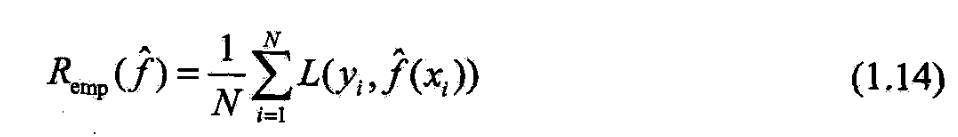测试误差是模型关于测试数据集的平均损失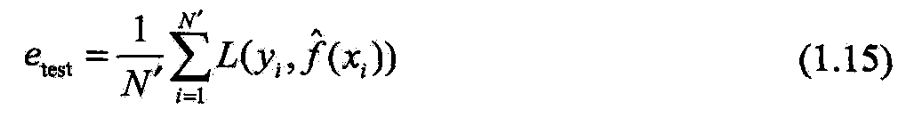损失函数是0-1损失时,测试误差就成测试数据集上的误差率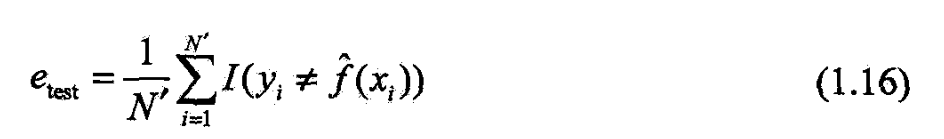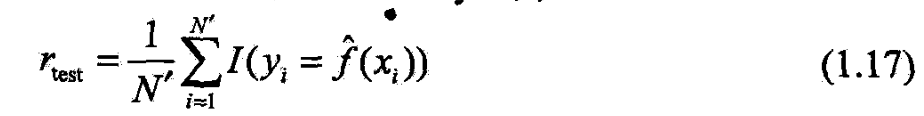训练误差的大小,对判断给定问题是不是一个容易学习的问题

是有意义的,但本质不重要

测试误差反映学习方法对未知的测试数据集的预测能力,
给定两种学习方法,

测试误差小的方法具有更好的预测能力,是更有效的

通常将学习方法对未知数据的预测能力称为泛化能カ

将在1.6节继续论

1.4.2过拟合与模型选择

当假设空间含不同复杂度(不同的参数个数)的模型时,就面临模型选择.
希望选择或学习一个合适的模型.
如果在假设空间中存在“真”模型,那么所选择的模型应该逼近真模型
具体地,所选择的模型要与真模型的参数个数相同,

所选择的模型的参数向量与真模型的参数向量相近

如果一味追求提高对训练数据的预测能力,

所选模型的复杂度往往比真模型更高.
称过拟合

过拟合指学习时选择的模型所含参数过多

以致出现这一模型对已知数据预测得很好,
但对未知数据预测很差

模型选择旨在避免过拟合并提高模型预测能力

给定一个训练集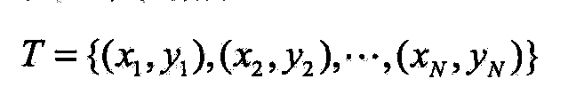设给定数据由$M$次多项式生成,选择最有可能产生这些数据的$M$次多项式,
即在$M$次多项式函数中选择一个对已知数据以及未知数据都有很好预测能力的

给定图1.2的10个数据点,用0~9次多项式对数据拟合

解决这一问题的方法可以这样
先确定模型复杂度,即确定多项式次数
给定模型复杂度下,按经验风险最小化,求解多项式系数
求以下经验风险最小化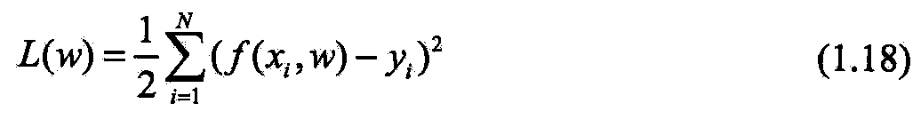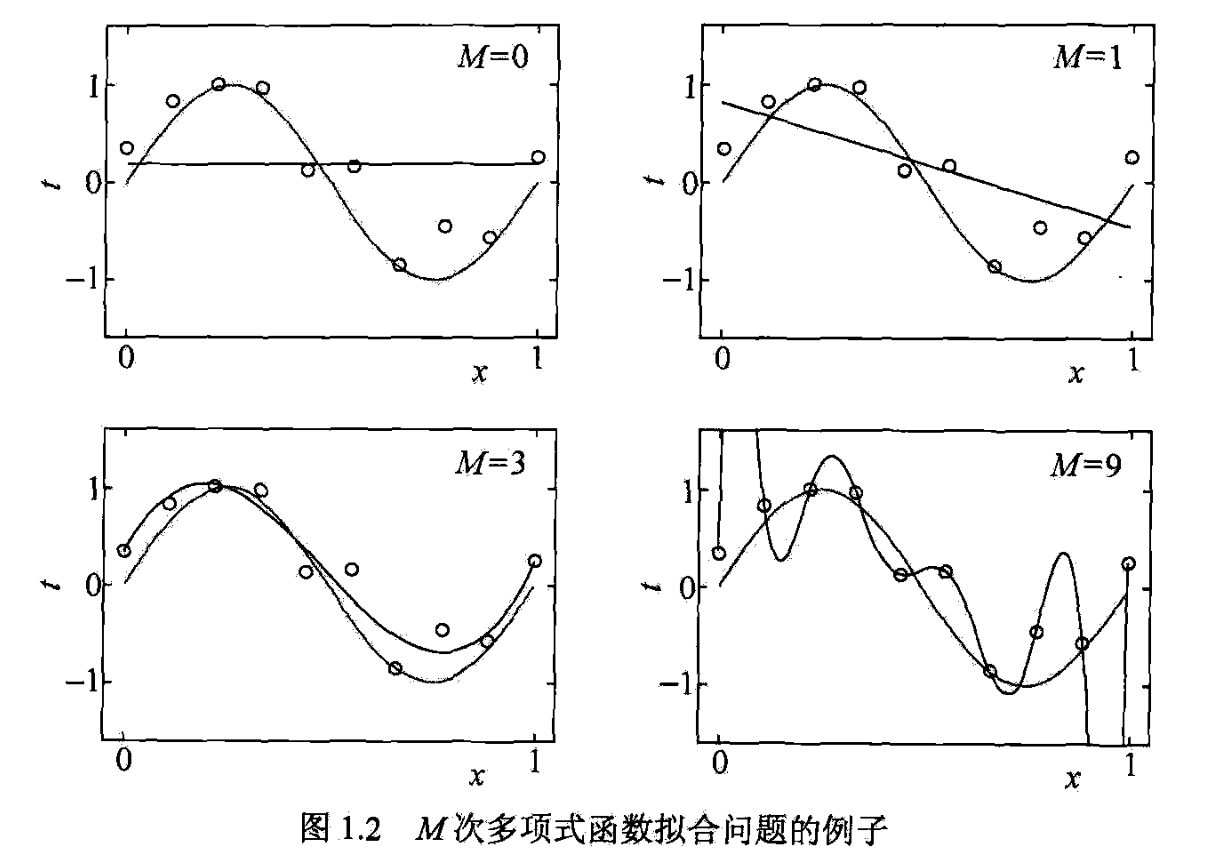将模型与训练数据代入(1.18)中,有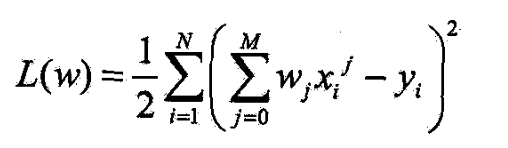对$w_j$求偏导并令其为0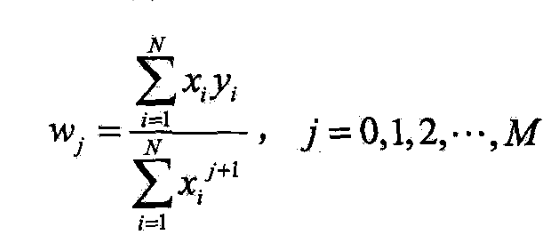=0,一个常数,数据拟合效果很差
=1,一条直线,也很差
M=9,多项式通过每个数据点,训练误差0
从对给定训练数据拟合的角度来说,效果最好
但因为训练数据本身存在噪声,这种拟合曲线对未知数据的预测能力并不最好,实际中不可取.过拟合发生
模型选择时,不仅要考虑对已知数据的预测能力

还要考虑对未知数据的预测能力.
=3时,多项式曲线对训练数据拟合效果足够好,模型也比较简单,是
一个较好的选择

随着多项式次数(模型复杂度)增加,训练误差会减小,直至趋向于0
测试误差随着多项式次数(模型复杂度)的增加先减后增
最终的目的是使测试误差最小.
多项式拟合中,就要选合适的多项式次数,以达到这一目的.
这结论对一般模型选择也成立

当模型复杂度增大时,训练误差逐渐减小并趋0
测试误差先减小,达到最小值后又增大
模型复杂度过大时,过拟合就发生
学习时就要防止过拟合,进行最优的模型选择,

即选择复杂度适当的模型,以达到测试误差最小

两种常用的模型选择方法:正则化与交叉验证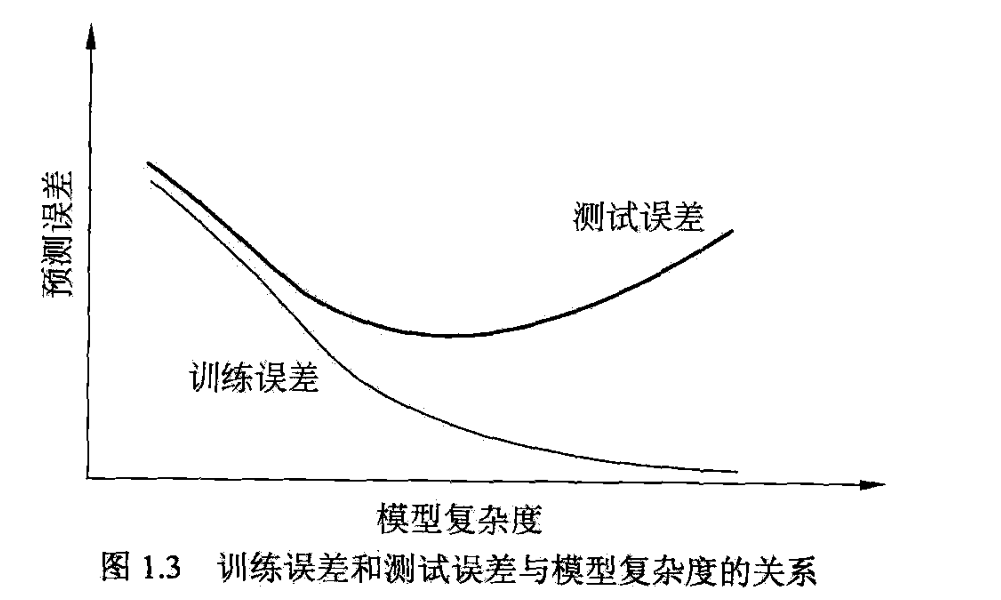1.5正则化与交叉验证
1.5.1正则化

模型选择的典型方法是regularization.
正则化是结构风险最小化策略的实现,

经验风险上加一个正则化项( regularizer)或罚项( penalty term),

一般模型越复杂,正则化值越大.
正则化项可以是模型参数向量的范数

正则化一般有如下形式
$\min_{f\in\mathcal{F}}\frac 1N\sum_{i=1}^NL(y_i,f(x_i))+\lambda J(f)\tag{1.19}$
经验风险,
正则化项,
$\lambda\ge 0$为调整两者之间关系的系数

正则化项可取不同形式.

回归问题中,损失函数是平方损失,正则化项可以是参数向量的$L_2$范数:
$L(w)=\frac 1N \sum_{i=1}^N(f(x_i;w)-y_i)^2+\frac \lambda 2||w||^2$

||w||$表示参数向量$w$的$L_2\$范

也可是参数向量的$L_1$范数
$L(w)=\frac 1N \sum_{i=1}^N(f(x_i;w)-y_i)^2+\lambda||w||_1$

$||w||_1$表示参数向量$w$的$L_1$

经验风险较小的模型较复杂(有多个非零参),
这时第2项的模型复杂度会较大.
正则化的作用是选择经验风险与模型复杂度同时较小的模型.

正则化符合Ocam’ s azor原理
在所有可能选择的模型中,能很好地解释已知数据且十分简单才是最好的
贝叶斯估计的角度看,

正则化项对应于模型的先验概率
可假设复杂的模型有较大先验概率,
简单的模型有较小的

1.5.2 交叉验证

另一种常用的模型选择方法是交叉验证

如果样本数据充足,模型选择的一种简单方法

是随机将数据集分三部分,训练集、验证集和测试集

训练集来训练模型
验证集用于模型的选择
测试集用于最终对学习方法的评估.
在学习到的模型中,

选择对验证集有最小预测误差的模型.
由于验证集有足够多的数据,用它对模型进行选择也是有效的

1.简单交叉验证
先随机将数据分两部分,
训练集,测试集(70%为训练集,30%为测试集)
然后用训练集在各种条件下(不同的参数个数)训练模型,

从而得到不同模型

在测试集上评价各个模型的测试误差,选出测试误差最小的

2.S折交叉验证
应用最多的是S折交叉验证,
先随机将已给数据切分为S个互不相交的大小相同的子集;然后用S-1个子集的数据训练模型,利用余下的子集测试;
将这一过程对可能的S种选择重复进行
最后选出S次评测中平均测试误差最小的

S折交叉验证特殊情形是S=N,称留一交叉验证,

数据缺乏的情况下使用,
N是给定数据集的容量

1.6 泛华能力
1.6.1 generalization ability

generalization ability

指该方法学习到的模型对未知数据的预测能力,

现实用最多的办法是通过测试误差来评价学习方法的泛化能力.

但此评价依赖测试数据集
测试数据集有限,得到的评价不可靠.

统计学习理论从理论上对学习方法的泛化能力分析

泛化误差定义.
学到的模型$\hat{f}$,
用这个模型对未知数据预测的误差

即generalization error
$R_{exp}(\hat{f})=E_P[L(Y,\hat{f}(X))]=\int_{\mathcal{X}\times \mathcal{Y}}L(y,\hat{f}(x))P(x,y)dxdy\tag{1.20}$

泛化误差反映学习方法的泛化能力,
如果一种方法学习的模型比另一种方法学习的模型有更小泛化误差,

则这种方法更有效.

泛化误差就

是所学习到的模型的期望风险.

1.6.2泛化误差上界

学习方法的泛化能力分析是通过研究泛化误差的概率上界进行的,

generalization error bound

通过比较两种学习方法的泛化误差上界比较优劣
泛化误差上界性质:

样本容量的函数,样本容量增加时,泛化上界趋于0
假设空间容量的函数,越大,模型越难学,泛化误差上界就越大

二类分类问题的泛化误差上界
二类分类问题
已知训练数据集$T=\{(x_1,y_1),(x_2,y_2),...,(x_N,y_N)\}$
它是从联合概率分布$P(X,Y)$独立同分布产生的,
$X∈R^n$,$Y\in\{-1,+1\}$.
假设空间是函数的有限集合$\mathcal{F}=\{f_1,f_2,...,f_d\}$

$d$是what?.

设$f$是从$\mathcal{F}$中选取的.

损失函数是0-1损失

$f$的期望风险和经验风险分别是
$R(f)=E[L(Y,f(X))]\tag{1.21}$
$\hat{R}(f)=\frac 1N \sum_{i=1}^NL(y_i,f(x_i))\tag{1.22}$
经验风险最小化函数是
$f_N=\argmin_{f\in \mathcal{F}}\hat{R}(f)\tag{1.23}$
人更关心$f_N$的泛化能力
$R(f_N)=E[L(Y,f_N(X))]\tag{1.24}$

从$\mathcal{F}=\{f_1,f_2,...,f_d\}$中选的$f$的泛化误差上界

定理1.1 泛化误差上界
对二分类,
设空间是有限集合$\mathcal{F}=\{f_1,f_2,...,f_d\}$
对任一$f\in\mathcal{F}$,
至少以概率$1-\delta$,不等式成立

$R(f)\le \hat{R}(f)+\varepsilon(d,N,\delta)\tag{1.25}$
$\varepsilon(d,N,\delta)=\sqrt{\frac{1}{2N}\left(logd+log\frac{1}{\delta}\right)}$

(1.25)$R(f)$是泛化误差,右端为泛化误差上界.

训练误差,其越小,泛化误差也越小,
$\varepsilon(d,N,\delta)$是N单减,N趋无穷趋0

也是$\sqrt{log d}$阶的函数
设空间$\mathcal{F}$包含的函数越多,其值越大

证明
Hoeffding不等式,

$S_n=\sum_{i=1}^nX_i$是独随$X_1,X_2,...,X_n$之和,都$\in[a,b]$,
则对$t>0$
成立:
$P(S_n-ES_n\ge t)\le exp\left(\frac{-2t^2}{\sum_{i=1}^n(b_i-a_i)^2}\right)\tag{1.27}$
$P(S_n-ES_n\le -t)\le exp\left(\frac{-2t^2}{\sum_{i=1}^n(b_i-a_i)^2}\right)\tag{1.28}$

对$\forall f\in \mathcal{F}$

$\hat{R}(f)$是$N$个独随$L(Y,f(X))$的样均,
$R(f)$是随变$(Y,f(X)$的期望.
若loss取[0,1],即$[a_i,b_i]=[0,1]$
由Hoeffding知,
对$\varepsilon>0$,
下成立:
$P(R(f)-\hat{R}(f)\ge \varepsilon)\le exp(-2N\varepsilon^2)$

由于谁是有限集合,

故
$P(\exists f\in \mathcal{F}:R(f)-\hat{R}(f)\ge \varepsilon$
$=P(\cup_{f\in\mathcal{F}}\{R(f)-\hat{R}(f)\ge \varepsilon\})$
$\le \sum_{f\in\mathcal{F}}P(R(f)-\hat{R}(f)\ge \varepsilon)$
$\le dexp(-2N\varepsilon^2)$
或等价,对$\forall \in\mathcal{F}$,有
$P(R(f)-\hat{R}(f)<\varepsilon)\ge 1-dexp(-2N\varepsilon^2)$
令
$\delta=dexp(-2N\varepsilon^2)\tag{1.29}$
则
$P(R(f)<\hat{R}(f)+\varepsilon)\ge 1-\delta$
即至少以概率$1-\delta$有
$R(f)<\hat{R}(f)+\varepsilon$
$\varepsilon$由(1.29)得到,即(1.26)

从泛化误差上界可知,
$R(f_N)<\hat{R}(f_N)+\varepsilon(d,N,\delta)$
(1,26),(1.23).
训练误差小的模型,其泛化误差也,

上讨论的是假设空间含有限个函数
对一般假设空间
要找到泛化误差界不这么简单,
这里不介绍

1.7 生成和判别model

监督学习的任务:

对给定的输入预测相应输出
模型一般形式: 决策函数
$Y=f(X)$

或条件概率分布
$P(Y|X)$

监督学习方法分：generative 和discriminative

生成方法由数据学习联合概率分布$P(X,Y)$
然后求出条件概率分布$P(Y|X)$作为预测的模型,
即生成模型:
$P(Y|X)=\frac{P(X,Y)}{P(X)}$
为啥叫生成方法,

模型表示了给定输入$X$产生输出$Y$的生成关系.

朴素贝叶斯法和HMM,

判别方法由数据直接学习决策函数$f(X)$或条件概率分布$P(Y|X)$作为预测的模型,即判别模型
判别方法关心的是对给定的输入$X$,应预测什么样输出$Y$.
k近邻、感知机、决策树、逻辑斯谛回归模型、最大熵模型、支持向量机、提升方法和条件随机场

监督学习中,生成和判别各有优缺点,适合于不同条件下的学习

特点:
可还原联合概率分布$P(X,Y)$,判别不能;
学习收敛速度更快,当样本容量增加的时候,学到的模型更快地收敛于真实模型;
存在隐变量时,仍可用生成方法,此时判别就不能用.

特点:
判别方法直接学习的是条件概率$P(Y|X)$或决策函数$f(X)$,直接面对预测,学习的准确率高;
由于直接学习$P(Y|X)$或$f(X)$,可以对数据进行各种程度上的抽象、定义特征并使用特征,因此可简化学习问题


展开全文• 22联合概率分布 23假设空间 3 统计学习的三要素 31 模型 32策略 4模型评估与模型选择 41训练误差与测试误差 42过拟合与模型选择 5正则化与交叉验证 51正则化 52交叉验证 6泛化能力 61泛化误差 7生成模型与判别模型 8...
统计学习方法

统计学习方法
一统计学习方法概论
1 统计学习
2监督学习
21输入空间特征空间输出空间
22联合概率分布
23假设空间

3 统计学习的三要素
31 模型
32策略

4模型评估与模型选择
41训练误差与测试误差
42过拟合与模型选择

5正则化与交叉验证
51正则化
52交叉验证

6泛化能力
61泛化误差

7生成模型与判别模型
8分类问题
9标注问题
10回归问题

一、统计学习方法概论

1.1 统计学习

统计学习的方法是基于数据构建统计模型从而对数据进行预测与分析。统计学习由以下几个主要组成部分：
- 监督学习（supervised learning）
- 非监督学习 （unsupervised learning）
- 半监督学习 （semi-supervised learning）
- 强化学习 （reinforcement learning）

Supervised learning is the machine learning task of inferring a function from labeled training data.

Unsupervised learning is the machine learning task of inferring a function to describe hidden structure from unlabeled data.
Wikipedia：
https://en.wikipedia.org/wiki/Supervised_learning
https://en.wikipedia.org/wiki/Unsupervised_learning

统计学习方法的三要素：
- 模型 （model）
- 策略 （strategy）
- 算法 （algorithm）

实现统计学习方法的步骤如下：
（1）得到一个有限的训练数据集合；
（2）确定包含所有可能的模型的假设空间，即学习模型的集合；
（3）确定模型选择的准则，即学习的策略；
（4）实现求解最有模型的算法，即学习的算法；
（5）通过学习方法选择最优模型；
（6）利用学习的最优模型对新数据进行预测或分析。

1.2监督学习

1.2.1.输入空间、特征空间、输出空间

在监督学习中，将输入与输出所有可能取值的集合分别称为输入空间（input space）与输出空间（output space）。
每个具体的输入是一个实例（instance），通常由特征向量（feature vector）表示，所有的特征向量存在的空间称为特征空间（feature space）。

1.2.2联合概率分布

监督学习假设输入与输出的随机变量X和Y遵循联合概率分布P(X,Y)$P(X,Y)$.
P(X,Y)$P(X,Y)$表示分布函数，或分布密度函数。

1.2.3假设空间

监督学习的目的在于学习一个由输入到输出的映射，这一映射由模型来表示。
换句话说，学习的目的就在于找到最好的这样的模型。
模型属于由输入空间到输出空间的映射的集合。这个集合就是假设空间（hypothesis space）。

1.3 统计学习的三要素

1.3.1 模型

模型的假设空间（hypothesis space）包含所有可能的条件概率分布或决策函数。
假设空间可以用 F 表示，可以定义为决策函数的集合,称为非概率模型
F={f|Y=f(X)} F=\{  f|Y=f(X) \}
也可以定义为条件概率的集合，称为概率模型：
F={P|P(Y|X)} F=\{ P|P(Y|X)\}

1.3.2策略

损失函数
监督学习问题是在假设空间F中选取模型f作为决策函数，对于给点的输入X，由f(x)$f(x)$给出相应的输出Y$Y$,输出的预测值与真实值Y$Y$之间错误的程度用损失函数（loss function）或代价函数（cost function）表示,通常有：
（1）0-1损失函数（0-1 loss function）
L(Y,f(X))=(Y−f(X))2L(Y,f(X))=(Y-f(X))^2
（3）绝对损失函数（absolute loss function）
L(Y,f(X))=|Y−f(X)|L(Y,f(X))=|Y-f(X)|
（4）对数损失函数（logarithmic loss function）
L(Y,P(Y|X)=−logP(Y|X)L(Y,P(Y|X)=-log P(Y|X)
风险函数
损失函数值越小，模型就越好。由于模型的输入，输出(X,Y)$(X,Y)$是随机变量，遵循联合分布P(X,Y)$P(X,Y)$，所以损失函数的期望就是：
Rexp(f)=Ep[L(Y,f(X)]=∫XxYL(y,f(x))P(x,y)dxdy R_ {exp}(f)=E_p[L(Y,f(X)]=\int_{X x Y}L(y,f(x))P(x,y)dxdy
这就是理论模型f(x)$f(x)$关于联合分布P(X,Y)$P(X,Y)$的平均意义下的损失，称为风险函数（risk function）或期望损失。
（1）经验风险：模型f(x)$f(x)$关于训练集的平均损失称为经验风险（empirical risk）或经验损失（empirical loss），记作Rexp$R_ {exp}$：
Rexp=1N∑i=1NL(yi,f(xi)) R_ {exp}=\frac {1}{N}\sum_{i=1}^{N}L(y_i,f(x_i))
（2）结构风险：结构风险在经验风险的基础上加上表示模型复杂度的正则化项（regularizer）或罚项（penalty term），其中J(f)$J(f)$为模型的复杂度，λ≥0$\lambda\ge0$是系数,用以权衡经验风险和模型复杂度。
Rrsm=1N∑i=1NL(yi,f(xi))+λJ(f) R_ {rsm}=\frac {1}{N}\sum_{i=1}^{N}L(y_i,f(x_i))+\lambda J(f)
经验风险最小化和结构风险最小化
经验风险最小化（empirical risk minimization，ERM）的策略认为，经验风险最小的模型就是最优的模型。
当样本容量足够大的时候，经验风险最小化能保证有很好的学习效果，在现实中被广泛采纳。比如，极大似然估计（maximum likelihood estimation）就是经验风险最小化的一个例子。
但是，当样本容量很小的时候，经验风险最小化学习的效果就未必很好，会产生过拟合（over-fitting）的现象。
结构风险最小化（structural risk minimization，SRM）是为了防止过拟合而提出的策略。结构风险最小化等价于正则化（regularization）。
贝叶斯估计中的最大后验概率估计（maximum posterior probability estimation，MAP）就是结构风险最小化的一个例子。

1.4模型评估与模型选择

1.4.1训练误差与测试误差

训练误差：假设学习到的模型是Y=f^(X)$Y=\hat f(X)$,训练误差是模型Y=f^(X)$Y=\hat f (X)$关于训练数据集的平均损失,其中N是训练样本容量：
Remp(f^)=1N∑i=1NL(yi,f^(xi))R_{emp}(\hat f)=\frac{1}{N}\sum_{i=1}^{N}L(y_i,\hat f (x_i))
测试误差：测试误差是模型Y=f^(X)$Y=\hat f(X)$关于测试数据集的平均损失,其中N’ 是测试样本容量：
etest(f^)=1N′∑i=1N′L(yi,f^(xi))e_{test}(\hat f)=\frac{1}{N'}\sum_{i=1}^{N'}L(y_i,\hat f (x_i))

1.4.2过拟合与模型选择

过拟合(over-fitting)：如果一味追求提高对训练数据的预测能力，所选的模型的复杂度则往往会比真的模型更高。过拟合是指的学习时选择的模型所包含的参数过多，以致于出现这一模型对已知数据预测得很好，但对未知数据预测得很差的现象。
模型选择旨在避免过拟合并提高模型的预测能力。

1.5正则化与交叉验证

1.5.1正则化

模型选择的典型方法是正则化（regularization）。正则化是结构风险最小化策略的实现，是在经验风险上加上一个正则化项（regularizer）或罚项（penalty term）。正则化项一般是模型复杂度的单调递增函数，模型越复杂，正则化值就越大。比如，正则化项可以是模型参数向量的范数。
正则化一般具有如下形式：
minf∈F1N∑i=1NL(yi,f(xi))+λJ(f)min_{f\in F} \frac{1}{N}\sum_{i=1}^{N}L(y_i,f(x_i))+\lambda J(f)
第一项是经验风险，第二项是正则化项, λ≥0$\lambda\ge 0$为调整两者之间关系的系数。
正则化可以取不同的形式：
- 例如，在回归问题中，损失函数是平方损失，正则化项可以是参数向量的L2$L_2$范数：
L(ω)=1N∑i=1N(f(xi;ω)−yi)2+λ2||ω||2L(\omega) =\frac{1}{N}\sum_{i=1}^{N}(f(x_i;\omega)-y_i)^2+\frac{\lambda}{2}||\omega||^2
这里||ω||$||\omega||$表示参数向量的L2$L_2$范数。

参考链接：http://blog.csdn.net/zouxy09/article/details/24971995
除了L1范数，还有一种更受宠幸的规则化范数是L2范数: ||W||2。它也不逊于L1范数，它有两个美称，在回归里面，有人把有它的回归叫“岭回归”（Ridge Regression），有人也叫它“权值衰减weight decay”。这用的很多吧，因为它的强大功效是改善机器学习里面一个非常重要的问题：过拟合。至于过拟合是什么，上面也解释了，就是模型训练时候的误差很小，但在测试的时候误差很大，也就是我们的模型复杂到可以拟合到我们的所有训练样本了，但在实际预测新的样本的时候，糟糕的一塌糊涂。

正则化项可以是参数向量的L1$L_1$范数：
L(ω)=1N∑i=1N(f(xi;ω)−yi)2+λ||ω||1L(\omega) =\frac{1}{N}\sum_{i=1}^{N}(f(x_i;\omega)-y_i)^2+\lambda||\omega||_1
这里||ω||1$||\omega||_1$表示参数向量ω$\omega$的L1$L_1$范数。

参考链接：http://blog.csdn.net/zouxy09/article/details/24971995
L0范数是指向量中非0的元素的个数。如果我们用L0范数来规则化一个参数矩阵W的话，就是希望W的大部分元素都是0。这太直观了，太露骨了吧，换句话说，让参数W是稀疏的。OK，看到了“稀疏”二字，大家都应该从当下风风火火的“压缩感知”和“稀疏编码”中醒悟过来，原来用的漫山遍野的“稀疏”就是通过这玩意来实现的。但你又开始怀疑了，是这样吗？看到的papers世界中，稀疏不是都通过L1范数来实现吗？脑海里是不是到处都是||W||1影子呀！几乎是抬头不见低头见。没错，这就是这节的题目把L0和L1放在一起的原因，因为他们有着某种不寻常的关系。那我们再来看看L1范数是什么？它为什么可以实现稀疏？为什么大家都用L1范数去实现稀疏，而不是L0范数呢？

L1范数是指向量中各个元素绝对值之和，也有个美称叫“稀疏规则算子”（Lasso regularization）。现在我们来分析下这个价值一个亿的问题：为什么L1范数会使权值稀疏？有人可能会这样给你回答“它是L0范数的最优凸近似”。实际上，还存在一个更美的回答：任何的规则化算子，如果他在Wi=0的地方不可微，并且可以分解为一个“求和”的形式，那么这个规则化算子就可以实现稀疏。这说是这么说，W的L1范数是绝对值，|w|在w=0处是不可微，但这还是不够直观。这里因为我们需要和L2范数进行对比分析。所以关于L1范数的直观理解，请待会看看第二节。

对了，上面还有一个问题：既然L0可以实现稀疏，为什么不用L0，而要用L1呢？个人理解一是因为L0范数很难优化求解（NP难问题），二是L1范数是L0范数的最优凸近似，而且它比L0范数要容易优化求解。所以大家才把目光和万千宠爱转于L1范数。

NP困难（NP-hard,non-deterministic polynomial-time hard）问题是计算复杂性理论中最重要的复杂性类之一。某个问题被称作NP困难，当所有NP问题可以在多项式时间图灵归约到这个问题。
NP困难参考链接：https://zh.wikipedia.org/wiki/NP%E5%9B%B0%E9%9A%BE

1.5.2交叉验证

另一种常见的模型选择方法是交叉验证（cross validation）。
如果给定的样本数据充足，进行模型选择的一种简单方法是随机的将数据集切分成三部分，分别为**训练集（training set），验证集（validation set）和测试集（test set）**。

1.6泛化能力

1.6.1泛化误差

**学习方法的泛化能力（generalization ability）是指由该方法学习到的模型对位置数据的预测能力**。
现实中采用最多的方法是通过测试误差来评价学习方法的泛化能力，但这种评价是依赖于测试数据集的。
- **泛化误差（generalization error）**：如果学习到的模型是f^$\hat f$,那么用这个模型对未知数据预测的误差即为泛化误差。
Rexp(f^)=Ep[L(Y,f^(X)]=∫XxYL(y,f^(x))P(x,y)dxdy R_ {exp}(\hat f)=E_p[L(Y,\hat f(X)]=\int_{X x Y}L(y,\hat f( x))P(x,y)dxdy
泛化误差反应了学习方法的泛化能力，如果一种方法学习的模型比另一种方法学习的模型具有更小的泛化误差，那么这种方法就更加有效。事实上，**泛化误差就是所学习到的模型的期望风险**。

1.7生成模型与判别模型

监督学习的任务就是学习一个模型，应用这一模型，对给定的输入预测相应的输出。这一模型的一般形式为决策函数：
Y=f(X)Y=f(X)
或者条件概率分布：
P(Y|X)P(Y|X)
-  监督学习方法又可以分为**生成方法（generative approach）**和**判别方法（discriminative approach）**。所学习的到模型分别称为**生成模型**（generative model）和**判别模型**（discriminative model）。

生成方法由数据学习联合概率分布P(X,Y)$P(X,Y)$,然后求出条件概率分布P(Y|X)$P(Y|X)$作为预测的模型， 即生成模型：
P(Y|X)=P(X,Y)P(X) P(Y|X)=\frac{P(X,Y)}{P(X)}
这样的方法之所有称为生成方法，是因为模型表示了给定输入X产生输出Y的生成关系。典型的生成模型有：朴素贝叶斯法和隐马尔可夫模型。
判别方法由数据直接学习决策函数f(X)$f(X)$或者条件概率分布P(Y|X)$P(Y|X)$作为预测的模型，即判别模型。判别方法关心的是对给定的输入X$X$，应该预测什么样的输出Y$Y$。典型的判别模型包括：k近邻法、感知机、决策树、逻辑回归模型、最大熵模型、支持向量机、提升方法和条件随机场。

1.8分类问题

对于二分类问题常用的指标是精确率（precision）与召回率（recall）。通常以关注的类为正类，其他类为负类，分类器在测试数据集上的预测正确与否可以分成4类：
- TP ——将正类预测为正类数
- FN——将正类预测为负类数
- FP——将负类预测为正类数
- TN——将负类预测为负类数
精准率定义为P=TPTP+FPP=\frac{TP}{TP+FP}
召回率定义为R=TPTP+FNR=\frac{TP}{TP+FN}

1.9标注问题

标注（tagging）也是一个监督学习问题。可以认为标注问题是分类问题的一个推广，标注问题又是更复杂的结构预测（structure prediction）问题的简单形式。标注问题的输入是一个观测序列，输出是一个标记序列或状态序列。标注问题的目标在于学习一个模型，使它能够对广策序列给出标记序列作为预测。
标注常用的统计学习方法有：隐马尔科夫模型，条件随机场。标记问题在信息抽取，自然语言处理等领域被广泛应用，是这些领域的基本问题。

1.10回归问题

回归（regression）是监督学习的另一个问题。回归用于预测输入变量（自变量）与输出变量（因变量）之间的关系，特别是当输入变量发生变化时，输出变量的值随之发生的变化。回归模型正是表示输入变量到输出变量之间映射的函数。回归问题的学习等价于函数拟合：选择一条函数曲线能使其很好地拟合已知数据且很好地预测未知数据。
回归学习最常用的损失函数是平方损失函数，在此情况下，回归问题可以由著名的最小二乘法（least square）求解。
展开全文机器学习
• 文章目录1.1 统计学习1.1.1 统计学习的特点1.1.2 统计学习的对象1.1.3 统计学习的目的1.1.4 统计学习的方法1.1.5 ...联合概率分布1.2.1.3 假设空间1.2.2 问题的形式化1.3 统计学习三要素1.3.1 模型1.3.2 策略1.3....
文章目录1.1 统计学习1.1.1 统计学习的特点1.1.2 统计学习的对象1.1.3 统计学习的目的1.1.4 统计学习的方法1.1.5 统计学习的研究1.1.6 统计学习的重要性1.2 监督学习1.2.1 基本概念1.2.1.1 输入空间、特征空间与输出空间1.2.1.2 联合概率分布1.2.1.3 假设空间1.2.2 问题的形式化1.3 统计学习三要素1.3.1 模型1.3.2 策略1.3.2.1 损失函数和风险函数1.3.3 算法1.4 模型评估与模型选择1.4.1 训练误差与测试误差1.4.2 过拟合与模型选择1.5 正则化与交叉验证1.5.1 正则化1.5.2 交叉验证1.6 生成模型与判别模型1.7 分类问题
刚开始学习这本教材时，看了第1章，说的内容看完基本上也懂，没啥具体的算法，也就没有做总结，现在整本书过了一遍，感觉第1章内容还是很重要的。整本书是一个体系的，那么这个学习记录肯定也不能缺少这一环。在学习过程中，看了不少博客，感觉严谨性上还是书本更好一些，当然博客中也有很多非常不错的内容。博客毕竟限于篇幅，在完整性和严谨性上稍有欠缺。后面学习新的知识还是以书本为主，博客、网络为辅，学习新的知识还是尽可能选择大众评价较高的教材，这样能减少挑选教材的成本。
1.1 统计学习
1.1.1 统计学习的特点
统计学习是关于计算机基于数据构建概率统计模型并运用模型对数据进行预测与分析的一门学科。也称为统计机器学习。
主要特点：

统计学习一计算机及网络为平台，是建立在计算机及网络之上的；
统计学习以数据为研究对象，是数据驱动的学科；
统计学习的目的是对数据进行预测与分析；
统计学习以方法为中心，统计学习方法构建模型并应用模型进行预测和分析；
统计学习是概率论、统计学、信息论、计算理论、最优化理论即计算机科学等多个领域的交叉学科，并且在发展中逐步形成独自的理论体系与方法论。

1.1.2 统计学习的对象
统计学习的对象是数据，它从数据出发，提取数据的特征，抽象出数据的模型，发现数据中的知识，又回到数据的分析与预测中去。
统计学习关于数据的基本假设是同类数据具有一定的统计规律性，这是统计学习的前提。
1.1.3 统计学习的目的
统计学习用于对数据进行预测与分析，特别是对未知数据进行预测与分析。对数据的预测可以使计算机更加智能化，对数据的分析可以让人们获取新的知识，给人们带来新的发现。
1.1.4 统计学习的方法
统计学习的方法是基于数据构建统计模型从而对数据进行预测与分析。统计学习由监督学习、非监督学习、半监督学习和强化学习等组成。
统计学习方法的步骤：

得到一个有限的训练数据集合；
确定包含所有可能的模型的假设空间，即学习模型的集合；
确定模型选择的准则，及学习的策略；
实现求解最优模型的算法，即学习的算法；
通过学习方法选择最优模型；
利用学习的最优模型对新数据进行预测或分析。

1.1.5 统计学习的研究
统计学习研究一般包括统计学习方法、统计学习理论及统计学习应用。

统计学习方法的研究旨在开发新的学习方法；
统计学习理论的研究在于探求统计学习方法的有效性与效率，以及统计学习的基本理论问题；
统计学习应用的研究主要考虑将统计学习方法应用到实际问题中去，解决实际问题。

1.1.6 统计学习的重要性

统计学习是处理海量数据的有效方法；
统计学习是计算机智能化的有效手段；
统计学习是计算机科学发展的一个重要组成部分。可以认为计算机科学由三维组成：系统、计算、信息。统计学习主要属于信息这一维，并在其中起着核心作用。

1.2 监督学习
监督学习的任务是学习一个模型，使模型能够对任意给定的输入，对其相应的输出做出一个好的预测（注意，这里的输入、输出是指某个系统的输入域输出，与学习的输入域输出不同）。计算机的基本操作就是给定一个输入产生一个输出，所以监督学习是极其重要的统计学习分支。
1.2.1 基本概念
1.2.1.1 输入空间、特征空间与输出空间
在监督学习中，将输入与输出所有可能取值的集合分别称为输入空间与输出空间。输入域输出空间可以是有限元素的集合，也可以是整个欧式空间。输入空间与输出空间可以是同一个空间，也可以是不同的空间；但通常输出空间远远小于输入空间。

特征向量：每个具体的输入是一个实例；
特征空间：特征向量存在的空间
输入空间有时与特征空间一致，有时不一致，不一致的话就将输入空间映射到特征空间，因为模型实际上是定义在特征空间上的。

回归问题：输入变量与输出变量均为连续变量的预测问题；
分类问题：输出变量为有限个离散变量的预测问题称为分类问题；
标注问题：输入变量与输出变量均为变量序列的预测问题。
1.2.1.2 联合概率分布
监督学习假设输入与输出的随机变量$X$和$Y$遵循联合概率分布$P(X,Y)$。在学习过程中，假设这一联合概率分布存在。训练数据与测试数据被看作是依据联合概率分布$P(X,Y)$独立同分布产生的。
1.2.1.3 假设空间
模型：输入空间到输出空间的映射；
假设空间：模型的集合。
1.2.2 问题的形式化在学习过程中，学习系统利用给定的训练数据集，通过学习（或训练）得到一个模型，表示为条件概率分布$\hat{P}(Y|X)$或决策函数$Y = \hat{f}(X)$。条件概率分布$\hat{P}(Y|X)$或决策函数$Y = \hat{f}(X)$描述输入与输出随机变量之间的映射关系。

预测过程中，预测系统对于给定的测试样本集中的 输入$x_{N+1}$，由模型$y_{N+1} = arg\max_{y_{N+1}}\,\hat{P}(y_{N+1}|x_{N+1})$或$y_{N+1} = \hat{f}(x_{N+1})$给出相应的输出$y_{n+1}$。

1.3 统计学习三要素
统计学习方法都是由模型、策略和算法构成的,即：
$方法 = 模型 + 策略 + 算法$
1.3.1 模型
模型就是所要学习的条件概率分布或决策函数。假设空间包含所有可能的条件概率分布或决策函数。
1.3.2 策略
有了假设空间，统计学习接着需要考虑的是按照什么样的准则学习或选择最优的模型。
1.3.2.1 损失函数和风险函数
损失函数度量模型一次预测的好坏，风险函数度量平均意义下模型预测的好坏。
损失函数度量预测错误的程度，损失函数是$f(X)$和$Y$的非负实值函数，记作$L(Y,f(X))$。
常用的损失函数：

0-1损失函数
$L(Y,f(X)) = \begin{cases} 1, & Y \not= f(X) \\[2ex] 0, & \text{Y = f(X)} \end{cases}$

平方损失函数
$L(Y,f(X)) = (Y- f(X))^2$

绝对损失函数
$L(Y,f(X)) = |Y - f(X)|$

对数损失函数或对数似然损失函数
$L(Y,P(Y|X)) = -logP(Y|X)$

损失函数的期望
$R_{exp}(f) = E_P[L(Y,f(X))] = \int_{\bf{X\times Y}}L(y,f(x))P(x,y)dxdy$
这是理论上模型$f(X)$关于联合分布$P(X,Y)$的平均意义下的损失，称为风险函数或期望损失。
学习的目标就是选择期望风险最小的模型。由于联合分布$P(X,Y)$是未知的，$R_{exp}(f)$不能直接计算。就成为了先有鸡还是先有蛋的问题。那就找个方法来估算，根据大数定律。
模型$f(X)$关于训练数据集的平均损失称为经验风险或经验损失，记作$R_{emp}$
$R_{emp}(f) = \dfrac{1}{N}\sum_{i=1}^{N}L(y_i,f(x_i))$
期望风险$R_{exp}(f)$是模型关于联合分布的期望损失，经验风险$R_{emp}(f)$是模型关于训练样本集的平均损失。根据大数定律，当样本容量$N$趋于无穷时，经验分享$R_{emp}(f)$趋于期望风险$R_{exp}(f)$。很自然的想法是用经验风险估算期望风险，但实际中数据量有限，经验风险最小化并不一定能选出最优的模型，防止模型过拟合，引入结构风险，即加了正则化的经验风险。
这样，监督学习问题就变成了经验风险或结构风险函数的最优化问题。
1.3.3 算法
最优化问题的具体计算方法。
常用的算法有：

梯度下降
改进的迭代尺度法IIS
序列最小最优化算法（SMO）
前向分步算法
EM算法

1.4 模型评估与模型选择
1.4.1 训练误差与测试误差
训练误差，即模型关于训练数据集的平均损失
$R_{emp}(\hat{f}) = \dfrac{1}{N}\sum_{i=1}^N L(y_i,\hat{f}(x_i))$

$N$是训练样本容量。

测试误差，即模型关于测试数据集的平均损失
$e_{test} = \dfrac{1}{N'}\sum_{i=1}^{N'}L(y_i,\hat{f}(x_i))$

$N'$是测试样本容量。

当损失函数是0-1损失时，测试误差就是测试数据集上的误差率。此时
$e_{test} = \dfrac{1}{N'}\sum_{i=1}^{N'} I(y_i = \hat{f}(x_i))$
测试误差反映了学习方法对未知的测试数据集的预测能力，是学习中的重要概念。通常将学习方法对未知数据的预测能力称为泛化能力。现实中采用最多的办法是通过测试误差来评价学习方法的泛化能力。
1.4.2 过拟合与模型选择
过拟合：模型在训练数据集上表现很好，在测试数据集上表现不好。
模型选择方法：正则化与交叉验证1.5 正则化与交叉验证
1.5.1 正则化
模型选择的典型方法是正则化。正则化是结构风险最小化策略的实现，是在经验风险上加一个正则化项。正则化一般具有如下形式：
$\min_{f\in\bf{F}} \dfrac{1}{N}\sum_{i=1}^N L(y_i,f(x_i)) + \lambda J(f)$
正则化符合奥卡姆剃刀原理。能解释已知数据并且简单的模型才是一个好的模型。
1.5.2 交叉验证
如果给定的样本数据充足，进行模型选择的一种简单方法是随机地将数据集切分为三部分，分别为训练集、验证集和测试集。

训练集用来训练模型
验证集用于模型的选择
测试集用于最终对学习方法的评估

在许多实际应用中数据是不充足的，为了选择好的模型，可以采用交叉验证方法。交叉验证的基本思想是重复地使用数据。

简单交叉验证：首先随机地将已给数据分为两部分，一部分作为训练集，另一部分作为测试集（例如，70%的数据为训练集，30%的数据为测试集）。然后用训练集在各种参数下训练模型，再用测试集选出误差最小的模型。

$S$折交叉验证：首先随机地将已给数据分为S个互不相交的大小相同的子集；然后利用S-1个子集的数据训练模型，利用余下的子集测试模型；将这一过程对可能的S种选择重复进行；最后选出S次评测中平均测试误差最小的模型。$S$折交叉验证实际中应用最多。

留一交叉验证：$S$折交叉验证的特殊情况$S = N$，称为留一交叉验证。往往在数据缺乏的情况下使用。

1.6 生成模型与判别模型

生成方法由数据学习联合概率分布$P(X,Y)$，然后求出条件概率分布$P(Y|X)$作为预测的模型，即生成模型：
$P(Y|X) = \dfrac{P(X,Y)}{P(X)}$

判别方法由数据直接学习决策函数$f(X)$或者条件概率分布$P(Y|X)$作为预测的模型，即判别模型。

生成方法与判别方法的区别：

生成方法可以还原出联合概率分布$P(X,Y)$，而判别方法不能；
生成方法的学习收敛速度更快，当样本容量增加的时候，学到的模型可以更快地收敛于真实模型；
判别方法直接学习的是条件概率$P(Y|X)$或决策函数$f(X)$，直接面对预测，往往学习的准确率更高。

1.7 分类问题
分类问题：输出变量为有限个离散变量的预测问题称为分类问题；
对于二类分类问题常用的评价指标是精确率（precision）与召回率（recall）。通常以关注的类为正类，其他类为负类。

$\;$
预测正类
预测负类

实际正类
TP
FN

实际负类
FP
TN

精确率$P$：挑出来的瓜多少真的是好瓜
$P = \dfrac{TP}{TP + FP}$
召回率$R$：好瓜被挑出来的概率
$R = \dfrac{TP}{TP + FN}$
二类分类问题常用的评价指标还有精确率$P$和召回率$R$的调和均值$F_1$：
$\dfrac{2}{F_1} = \dfrac{1}{P} +\dfrac{1}{R}$
参考资料

李航 统计学习方法


展开全文统计学习方法
• 基本概念联合概率分布与假设空间2.问题的形式化 1.1统计学习(statistical learning) 本书讨论监督学习 1.统计学习的特点 定义：是关于计算机基于数据构建概论统计模型并运用模型对数据进行预测与分析的一门...机器学习 统计学习方法
• 输入X和输出Y具有联合分布概论的假设是监督学习关于数据的基本假设。 输入空间 特征空间 假设空间 ：监督学习的目的在于学习一个由输入到输出的映射，这一映射由模型来表示，模型属于由输入空间到输出空间的...
• 监督学习的假设是训练数据和测试数据是依联合概率分布P(X,Y)独立同分布产生的，独立同分布就是比如随机变量（X1,Y1）与（X2,Y2）具有相同的概率分布，并且取值相互独立。比如实验条件保持不变，一系列的抛硬币的正...机器学习 统计学
• 1、统计学习：监督学习、非监督学习、...2、监督学习 输入空间、输出空间、特征空间 联合概率分布、假设空间：输入空间到输出空间的映射的集合 3、三要素 3.1 模型 对监督学习，就是条件概率分布或决策函数 3.2 策略
• 多维随机变量及其分布 ... F(X,Y)为随机变量(X,Y)的分布函数，也称为随机变量X和Y的联合分布函数。 离散型的随机变量（X,Y）的分布律也叫随机变量X,Y的联合分布律。 连续型的二维随机变量有...
• 关于统计学习方法的知识，参考书《统计学习方法》，李航著，清华大学出版社。 ...第一章 统计学习方法概论 ...监督学习学到的模型又可以划分为生成模型...生成方法由数据学习联合概率分布P(X, Y)，然后求出生成模型 统计学
• 1.2 浅见目的第一章 统计学习及监督学习概论1.1 统计学习统计学习是什么，做什么的统计学习的对象统计学习的目的统计学习的方法1.2 统计学习的分类基本分类监督学习输入空间，输出空间，特征空间联合概率分布假设...统计学习方法 机器学习 深度学习
• 总结：本章主要是介绍一些基本的概念，起到统揽全书的作业。 1.1 统计学习 统计学习是基于数据构建概率模型并运用模型对数据进行预测分析的学科。 统计学习由监督学习、...联合概率分布：P(x,y)表示。举个例子，...统计学习方法
• 期望风险函数:模型关于联合分布的期望损失: 经验风险函数:模型关于训练样本集的平均损失: 2.3)经验风险最小化与结构风险最小化 经验风险最小化的策略认为:经验风险最小的模型就是最优的模...统计学习方法
• 第一章开始的话一、统计学习 [1.1]（一）总定义（二）统计学习的方法二、监督学习[1.2]（一）基本概念[1.2.1]1、输入空间、特征空间与输出空间2、联合概率分布3、假设空间（上面名词解释里面有讲）（二）问题的形式...统计学习方法 机器学习
• X和Y具有联合概率分布，训练数据与测试数据按照联合概率分布P(X,Y)独立同分布产生。 模型的假设空间是什么？ 模型属于输入空间到输出空间映射的集合，这个集合就是假设空间，假设空间的确定意味着学习范围的...
• 联合分布可以完全决定单个变量的分布，该分布称为边缘分布。 固定单一的列Y = yj ,以当前列的概率为基础，各个Pij 的等比例变换即为条件概率 pi∣j=P(X=xi∣Y=yj)=P(X=xi,Y=yj)P(Y=yj)=pijp⋅j,i=1,2,… p_{i | j}...统计学习方法
• 3.2 联合概率分布 I.监督学习假设输入与输出的随机变量X，Y遵循联合概率分布P(X,Y) II.训练和测试数据看作是P(X,Y)独立同分布产生的 3.3 假设空间 I.监督学习的目的在于学习一个由输入到输出的映射，这一映射机器学习
• 第一章 概论 统计学习的目的：用已知数据的信息来对未知数据进行预测和分析 统计学习方法： 监督（主要是对此讲解）...也就是输入实例的特征向量X，输出结果Y，假设X，Y遵循联合概率分布P(X,Y)-->为分布密度函数 训统计机器学习
• 统计学习方法概论 统计学习 统计学习是关于计算机基于数据构建统计模型并运用模型对数据... 联合概率分布监督学习假设输入和输出的随机变量X和Y遵循联合概率分 P(x,y)P(x,y).统计学习假设数据存在一定的统计规律，X和Y机器学习 统计学
• 第一章统计学习方法概论1.1 统计学习统计学习的主要特点是：统计学习的对象：统计学习的目的：统计学习的方法：实现一个统计学习方法的步骤如下：1.2 监督学习联合概率分布：假设空间：1.3 统计学习三要素统计学习...
• 第1章 统计学习方法概论监督学习统计学习三要素模型策略（经验风险和结构经验风险）判别模型与生成模型补充（含课后...监督学习有一个重要的假设：设输入的随机变量XXX和YYY遵循联合概率分布P(X,Y)P\left( {X,Y} \...
• 期望风险是模型关于联合分布的期望损失，经验风险是模型关于训练样本集的平均损失。根据大数定律，当样本容量N趋于无穷时，经验风险趋于期望风险。 经验风险最小化等价于极大似然估计。 结构风险最小化是为了防止...
• 统计学习方法概论学习统计学习统计学习的特点统计学习的目的统计学习的方法监督学习基本概念输入空间、特征空间与输出空间联合概率分布假设空间统计学习三要素模型策略损失函数和风险函数损失函数风险函数经验风险...机器学习 统计学
• 监督学习的基本假设：X和Y具有联合分布，即X和Y存在一定的统计规律。 输入变量与输出变量均为连续变量的预测问题称为回归问题； 输出变量为有限个离散变量的预测问题成为分类问题； 输入变量与输出变量均为变量...
• 第三单元：地理信息系统基础概论及GIS数字地图与遥感制图 主讲人：GIS硕士、南京路川公司专业技术开发工程师 第四单元：国际最优秀GIS最新技术应用---ARCGIS9.2/9.3系列专题 1、介绍目标前国际上最优秀的GIS软件...